Definition of Raman Spectroscopy

What is Raman Spectroscopy?

Raman spectroscopy is the measurement of the wavelength and intensity of inelastically scattered light from molecules.

The Raman scattered light occurs at wavelengths that are shifted from the incident light by the energies of molecular vibrations.

The mechanism of Raman scattering is different from that of infrared absorption, and Raman and IR spectra provide complementary information.

Typical applications are in structure determination, multicomponent qualitative analysis, and quantitative analysis.

Theory

The Raman scattering transition moment is: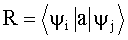where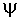i andj are the initial and final states, respectively, and a is the polarizability of the molecule: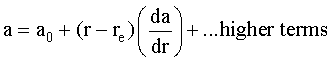where r is the distance between atoms and ao is the polarizability at the equilibrium bond length, re.

Polarizability can be defined as the ease of which an electron cloud can be distorted by an external electric field.

Since ao is a constant and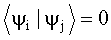R simplifies to: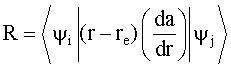The result is that there must be a change in polarizability during the vibration for that vibration to inelastically scatter radiation.

Examples of Raman active and inactive vibrations in CO2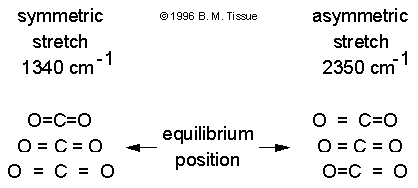The polarizability depends on how tightly the electrons are bound to the nuclei. In the symmetric stretch the strength of electron binding is different between the minimum and maximum internuclear distances.

Therefore the polarizability changes during the vibration and this vibrational mode scatters Raman light (the vibration is Raman active).

In the asymmetric stretch the electrons are more easily polarized in the bond that expands but are less easily polarized in the bond that compresses.

There is no overall change in polarizability and the asymmetric stretch is Raman inactive.

Raman line intensities are proportional to: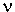4 *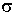() * I * exp(-Ei/kT) * C whereis the frequency of the incident radiation,() is the Raman cross section (typically 10-29 cm2), I is the radiation intensity, exp(-Ei/kT) is the Boltzmann factor for state i, and C is the analyte concentration.

Instrumentation

The most common light source in Raman spectroscopy is an Ar-ion laser.

Resonance Raman spectroscopy requires tunable radiation and sources are Ar-ion-laser-pumped cw dye lasers, or high-repetition-rate excimer-laser-pumped pulsed dye lasers.

Because Raman scattering is a weak process, a key requirement to obtain Raman spectra is that the spectrometer provide a high rejection of scattered laser light.

New methods such as very narrow rejection filters and Fourier-transform techniques are becoming more widespread.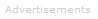Search the Dictionary for More Terms